# 2nd PUC Maths Question Bank Chapter 7 Integrals Ex 7.9

Students can Download Maths Chapter 7 Integrals Ex 7.9 Questions and Answers, Notes Pdf, 2nd PUC Maths Question Bank with Answers helps you to revise the complete Karnataka State Board Syllabus and score more marks in your examinations.

## Karnataka 2nd PUC Maths Question Bank Chapter 7 Integrals Ex 7.9

### 2nd PUC Maths Integrals NCERT Text Book Questions and Answers Ex 7.9

Question 1.
$$\int_{-1}^{1}(x+1) d x$$Question 2.
$$\int_{2}^{3} \frac{1}{x} d x$$Question 3.
$$\int_{1}^{2}\left(4 x^{3}-5 x^{2}+6 x+9\right) d x$$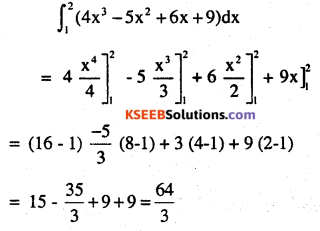Question 4.
$$\int_{0}^{\frac{\pi}{4}} \sin 2 x d x$$Question 5.
$$\int_{0}^{\frac{\pi}{2}} \cos 2 x d x$$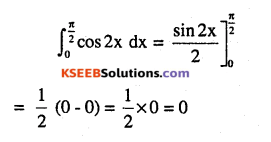Question 6.
$$\int_{4}^{5} e^{x} d x$$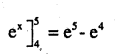Question 7.
$$\int_{0}^{\frac{\pi}{4}} \tan x d x$$Question 8.
$$\int_{\frac{\pi}{6}}^{\frac{\pi}{4}} \csc x d x$$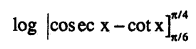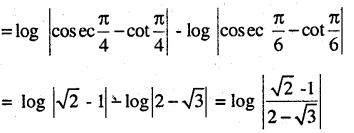Question 9.
$$\int_{0}^{1} \frac{d x}{\sqrt{1-x^{2}}}$$Question 10.
$$\int_{0}^{1} \frac{d x}{1+x^{2}}$$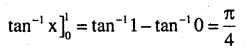Question 11.
$$\int_{2}^{3} \frac{d x}{x^{2}-1}$$Question 12.
$$\int_{0}^{\frac{\pi}{2}} \cos ^{2} x d x$$Question 13.
$$\int_{2}^{3} \frac{x d x}{x^{2}+1}$$Question 14.
$$\int_{0}^{1} \frac{2 x+3}{5 x^{2}+1} d x$$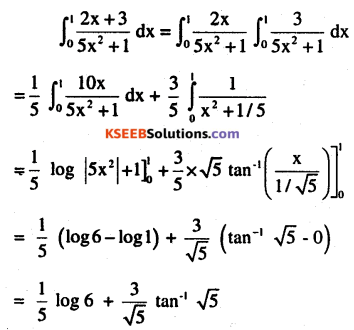Question 15.
$$\int_{0}^{1} x e^{x^{2}} d x$$Question 16.
$$\int_{1}^{2} \frac{5 x^{2}}{x^{2}+4 x+3}$$Question 17.
$$\int_{0}^{\frac{\pi}{4}}\left(2 \sec ^{2} x+x^{3}+2\right) d x$$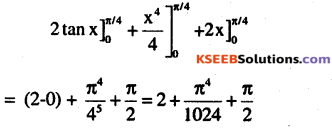Question 18.
$$\int_{0}^{\pi}\left(\sin ^{2} \frac{x}{2}-\cos ^{2} \frac{x}{2}\right) d x$$Question 19.
$$\int_{0}^{2} \frac{6 x+3}{x^{2}+4} d x$$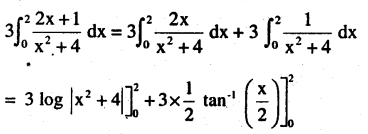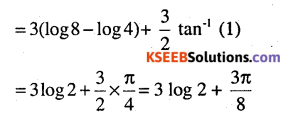Question 20.
$$\int_{0}^{1}\left(x e^{x}+\sin \frac{\pi x}{4}\right) d x$$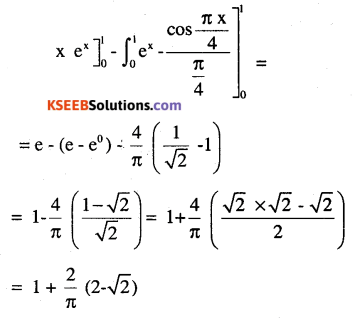Choose the correct answer in Exercise 21 and 22

Question 21.
$$\int_{1}^{\sqrt{3}} \frac{d x}{1+x^{2}} \text { equals }$$
(A) $$\frac{\pi}{3}$$
(B) $$\frac{2 \pi}{3}$$
(C) $$\frac{\pi}{6}$$
(D) $$\frac{\pi}{12}$$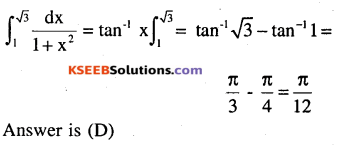$$\int_{0}^{\frac{2}{3}} \frac{d x}{4+9 x^{2}} \text { equals }$$
(A) $$\frac{\pi}{6}$$
(B) $$\frac{\pi}{12}$$
(C) $$\frac{\pi}{24}$$
(D) $$\frac{\pi}{4}$$